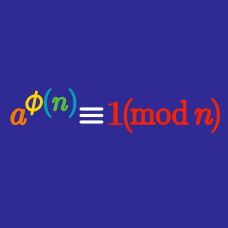Number Theory

# Finding the last few digits of a power - Euler's Theorem

What is the last digit of $13^{13}$?

What is the last digit of $54^{32}$?

What are the last two digits of $3^{45}$?

What are the last three digits of $6^{600}$?

What is the last digit of $22^{88}$?

×